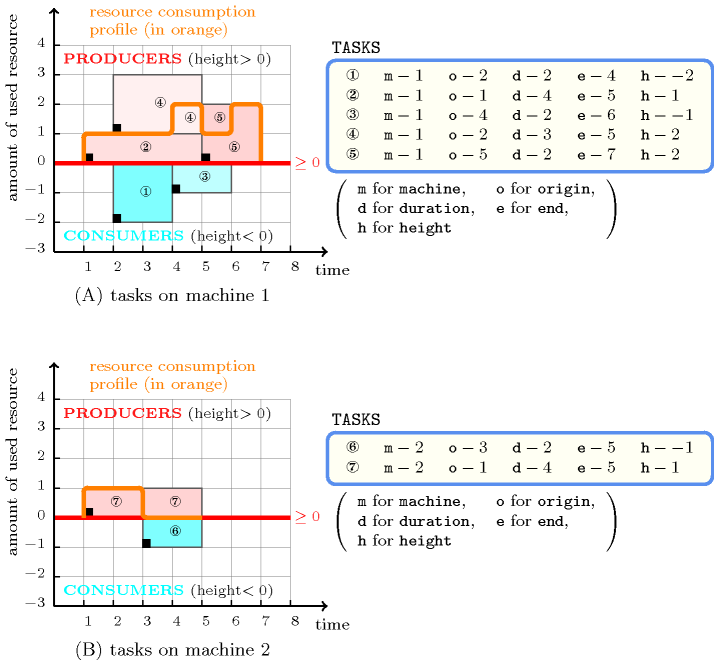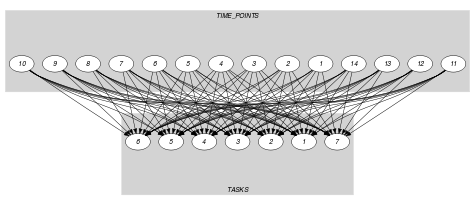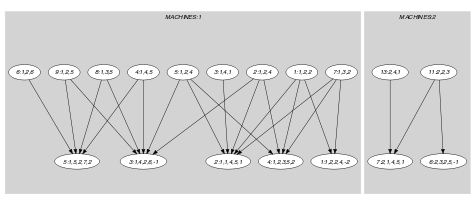## 5.101. cumulatives

Origin
Constraint

$\mathrm{𝚌𝚞𝚖𝚞𝚕𝚊𝚝𝚒𝚟𝚎𝚜}\left(\mathrm{𝚃𝙰𝚂𝙺𝚂},\mathrm{𝙼𝙰𝙲𝙷𝙸𝙽𝙴𝚂},\mathrm{𝙲𝚃𝚁}\right)$

Arguments
 $\mathrm{𝚃𝙰𝚂𝙺𝚂}$ $\mathrm{𝚌𝚘𝚕𝚕𝚎𝚌𝚝𝚒𝚘𝚗}\left(\begin{array}{c}\mathrm{𝚖𝚊𝚌𝚑𝚒𝚗𝚎}-\mathrm{𝚍𝚟𝚊𝚛},\hfill \\ \mathrm{𝚘𝚛𝚒𝚐𝚒𝚗}-\mathrm{𝚍𝚟𝚊𝚛},\hfill \\ \mathrm{𝚍𝚞𝚛𝚊𝚝𝚒𝚘𝚗}-\mathrm{𝚍𝚟𝚊𝚛},\hfill \\ \mathrm{𝚎𝚗𝚍}-\mathrm{𝚍𝚟𝚊𝚛},\hfill \\ \mathrm{𝚑𝚎𝚒𝚐𝚑𝚝}-\mathrm{𝚍𝚟𝚊𝚛}\hfill \end{array}\right)$ $\mathrm{𝙼𝙰𝙲𝙷𝙸𝙽𝙴𝚂}$ $\mathrm{𝚌𝚘𝚕𝚕𝚎𝚌𝚝𝚒𝚘𝚗}\left(\mathrm{𝚒𝚍}-\mathrm{𝚒𝚗𝚝},\mathrm{𝚌𝚊𝚙𝚊𝚌𝚒𝚝𝚢}-\mathrm{𝚒𝚗𝚝}\right)$ $\mathrm{𝙲𝚃𝚁}$ $\mathrm{𝚊𝚝𝚘𝚖}$
Restrictions
 $\mathrm{𝚛𝚎𝚚𝚞𝚒𝚛𝚎𝚍}$$\left(\mathrm{𝚃𝙰𝚂𝙺𝚂},\left[\mathrm{𝚖𝚊𝚌𝚑𝚒𝚗𝚎},\mathrm{𝚑𝚎𝚒𝚐𝚑𝚝}\right]\right)$ $\mathrm{𝚛𝚎𝚚𝚞𝚒𝚛𝚎}_\mathrm{𝚊𝚝}_\mathrm{𝚕𝚎𝚊𝚜𝚝}$$\left(2,\mathrm{𝚃𝙰𝚂𝙺𝚂},\left[\mathrm{𝚘𝚛𝚒𝚐𝚒𝚗},\mathrm{𝚍𝚞𝚛𝚊𝚝𝚒𝚘𝚗},\mathrm{𝚎𝚗𝚍}\right]\right)$ $\mathrm{𝚒𝚗}_\mathrm{𝚊𝚝𝚝𝚛}$$\left(\mathrm{𝚃𝙰𝚂𝙺𝚂},\mathrm{𝚖𝚊𝚌𝚑𝚒𝚗𝚎},\mathrm{𝙼𝙰𝙲𝙷𝙸𝙽𝙴𝚂},\mathrm{𝚒𝚍}\right)$ $\mathrm{𝚃𝙰𝚂𝙺𝚂}.\mathrm{𝚍𝚞𝚛𝚊𝚝𝚒𝚘𝚗}\ge 0$ $\mathrm{𝚃𝙰𝚂𝙺𝚂}.\mathrm{𝚘𝚛𝚒𝚐𝚒𝚗}\le \mathrm{𝚃𝙰𝚂𝙺𝚂}.\mathrm{𝚎𝚗𝚍}$ $|\mathrm{𝙼𝙰𝙲𝙷𝙸𝙽𝙴𝚂}|>0$ $\mathrm{𝚛𝚎𝚚𝚞𝚒𝚛𝚎𝚍}$$\left(\mathrm{𝙼𝙰𝙲𝙷𝙸𝙽𝙴𝚂},\left[\mathrm{𝚒𝚍},\mathrm{𝚌𝚊𝚙𝚊𝚌𝚒𝚝𝚢}\right]\right)$ $\mathrm{𝚍𝚒𝚜𝚝𝚒𝚗𝚌𝚝}$$\left(\mathrm{𝙼𝙰𝙲𝙷𝙸𝙽𝙴𝚂},\mathrm{𝚒𝚍}\right)$ $\mathrm{𝙲𝚃𝚁}\in \left[\le ,\ge \right]$
Purpose

Consider a set $𝒯$ of tasks described by the $\mathrm{𝚃𝙰𝚂𝙺𝚂}$ collection. When $\mathrm{𝙲𝚃𝚁}$ is equal to $\le$ (respectively $\ge$), the $\mathrm{𝚌𝚞𝚖𝚞𝚕𝚊𝚝𝚒𝚟𝚎𝚜}$ constraint forces the following condition for each machine $m$: At each point in time, where at least one task assigned on machine $m$ is present, the cumulated height of the set of tasks that both overlap that point and are assigned to machine $m$ should be less than or equal to (respectively greater than or equal to) the capacity associated with machine $m$. A task overlaps a point $i$ if and only if (1) its origin is less than or equal to $i$, and (2) its end is strictly greater than $i$. It also imposes for each task of $𝒯$ the constraint $\mathrm{𝚘𝚛𝚒𝚐𝚒𝚗}+\mathrm{𝚍𝚞𝚛𝚊𝚝𝚒𝚘𝚗}=\mathrm{𝚎𝚗𝚍}$.

Example
$\left(\begin{array}{c}〈\begin{array}{ccccc}\mathrm{𝚖𝚊𝚌𝚑𝚒𝚗𝚎}-1\hfill & \mathrm{𝚘𝚛𝚒𝚐𝚒𝚗}-2\hfill & \mathrm{𝚍𝚞𝚛𝚊𝚝𝚒𝚘𝚗}-2\hfill & \mathrm{𝚎𝚗𝚍}-4\hfill & \mathrm{𝚑𝚎𝚒𝚐𝚑𝚝}--2,\hfill \\ \mathrm{𝚖𝚊𝚌𝚑𝚒𝚗𝚎}-1\hfill & \mathrm{𝚘𝚛𝚒𝚐𝚒𝚗}-1\hfill & \mathrm{𝚍𝚞𝚛𝚊𝚝𝚒𝚘𝚗}-4\hfill & \mathrm{𝚎𝚗𝚍}-5\hfill & \mathrm{𝚑𝚎𝚒𝚐𝚑𝚝}-1,\hfill \\ \mathrm{𝚖𝚊𝚌𝚑𝚒𝚗𝚎}-1\hfill & \mathrm{𝚘𝚛𝚒𝚐𝚒𝚗}-4\hfill & \mathrm{𝚍𝚞𝚛𝚊𝚝𝚒𝚘𝚗}-2\hfill & \mathrm{𝚎𝚗𝚍}-6\hfill & \mathrm{𝚑𝚎𝚒𝚐𝚑𝚝}--1,\hfill \\ \mathrm{𝚖𝚊𝚌𝚑𝚒𝚗𝚎}-1\hfill & \mathrm{𝚘𝚛𝚒𝚐𝚒𝚗}-2\hfill & \mathrm{𝚍𝚞𝚛𝚊𝚝𝚒𝚘𝚗}-3\hfill & \mathrm{𝚎𝚗𝚍}-5\hfill & \mathrm{𝚑𝚎𝚒𝚐𝚑𝚝}-2,\hfill \\ \mathrm{𝚖𝚊𝚌𝚑𝚒𝚗𝚎}-1\hfill & \mathrm{𝚘𝚛𝚒𝚐𝚒𝚗}-5\hfill & \mathrm{𝚍𝚞𝚛𝚊𝚝𝚒𝚘𝚗}-2\hfill & \mathrm{𝚎𝚗𝚍}-7\hfill & \mathrm{𝚑𝚎𝚒𝚐𝚑𝚝}-2,\hfill \\ \mathrm{𝚖𝚊𝚌𝚑𝚒𝚗𝚎}-2\hfill & \mathrm{𝚘𝚛𝚒𝚐𝚒𝚗}-3\hfill & \mathrm{𝚍𝚞𝚛𝚊𝚝𝚒𝚘𝚗}-2\hfill & \mathrm{𝚎𝚗𝚍}-5\hfill & \mathrm{𝚑𝚎𝚒𝚐𝚑𝚝}--1,\hfill \\ \mathrm{𝚖𝚊𝚌𝚑𝚒𝚗𝚎}-2\hfill & \mathrm{𝚘𝚛𝚒𝚐𝚒𝚗}-1\hfill & \mathrm{𝚍𝚞𝚛𝚊𝚝𝚒𝚘𝚗}-4\hfill & \mathrm{𝚎𝚗𝚍}-5\hfill & \mathrm{𝚑𝚎𝚒𝚐𝚑𝚝}-1\hfill \end{array}〉,\hfill \\ 〈\mathrm{𝚒𝚍}-1\mathrm{𝚌𝚊𝚙𝚊𝚌𝚒𝚝𝚢}-0,\mathrm{𝚒𝚍}-2\mathrm{𝚌𝚊𝚙𝚊𝚌𝚒𝚝𝚢}-0〉,\ge \hfill \end{array}\right)$

Figure 5.101.1 shows with a thick line the cumulated profile on the two machines described by the $\mathrm{𝙼𝙰𝙲𝙷𝙸𝙽𝙴𝚂}$ collection. Within this profile a task with a positive (respectively negative) height is represented by a pink (respectively blue) rectangle, where the length of the rectangle corresponds to the duration of the task. The $\mathrm{𝚌𝚞𝚖𝚞𝚕𝚊𝚝𝚒𝚟𝚎𝚜}$ constraint holds since, both on machines 1 and 2, we have that at each point in time the cumulated resource consumption is greater than or equal to the limit 0 enforced by the last argument (i.e., the attribute $\mathrm{𝚌𝚊𝚙𝚊𝚌𝚒𝚝𝚢}$ of the items of the $\mathrm{𝙼𝙰𝙲𝙷𝙸𝙽𝙴𝚂}$ collection) of the $\mathrm{𝚌𝚞𝚖𝚞𝚕𝚊𝚝𝚒𝚟𝚎𝚜}$ constraint (i.e., we have a limit of 0 both on machines 1 and 2).

##### Figure 5.101.1. Resource consumption profiles on the different machines for the tasks of the Example slotTypical
 $|\mathrm{𝚃𝙰𝚂𝙺𝚂}|>1$ $\mathrm{𝚛𝚊𝚗𝚐𝚎}$$\left(\mathrm{𝚃𝙰𝚂𝙺𝚂}.\mathrm{𝚖𝚊𝚌𝚑𝚒𝚗𝚎}\right)>1$ $\mathrm{𝚛𝚊𝚗𝚐𝚎}$$\left(\mathrm{𝚃𝙰𝚂𝙺𝚂}.\mathrm{𝚘𝚛𝚒𝚐𝚒𝚗}\right)>1$ $\mathrm{𝚛𝚊𝚗𝚐𝚎}$$\left(\mathrm{𝚃𝙰𝚂𝙺𝚂}.\mathrm{𝚍𝚞𝚛𝚊𝚝𝚒𝚘𝚗}\right)>1$ $\mathrm{𝚛𝚊𝚗𝚐𝚎}$$\left(\mathrm{𝚃𝙰𝚂𝙺𝚂}.\mathrm{𝚎𝚗𝚍}\right)>1$ $\mathrm{𝚛𝚊𝚗𝚐𝚎}$$\left(\mathrm{𝚃𝙰𝚂𝙺𝚂}.\mathrm{𝚑𝚎𝚒𝚐𝚑𝚝}\right)>1$ $\mathrm{𝚃𝙰𝚂𝙺𝚂}.\mathrm{𝚍𝚞𝚛𝚊𝚝𝚒𝚘𝚗}>0$ $\mathrm{𝚃𝙰𝚂𝙺𝚂}.\mathrm{𝚑𝚎𝚒𝚐𝚑𝚝}\ne 0$ $|\mathrm{𝙼𝙰𝙲𝙷𝙸𝙽𝙴𝚂}|>1$ $\mathrm{𝙼𝙰𝙲𝙷𝙸𝙽𝙴𝚂}.\mathrm{𝚌𝚊𝚙𝚊𝚌𝚒𝚝𝚢}<$$\mathrm{𝚜𝚞𝚖}$$\left(\mathrm{𝚃𝙰𝚂𝙺𝚂}.\mathrm{𝚑𝚎𝚒𝚐𝚑𝚝}\right)$ $|\mathrm{𝚃𝙰𝚂𝙺𝚂}|>|\mathrm{𝙼𝙰𝙲𝙷𝙸𝙽𝙴𝚂}|$
Symmetries
• Items of $\mathrm{𝚃𝙰𝚂𝙺𝚂}$ are permutable.

• Items of $\mathrm{𝙼𝙰𝙲𝙷𝙸𝙽𝙴𝚂}$ are permutable.

• All occurrences of two distinct values in $\mathrm{𝚃𝙰𝚂𝙺𝚂}.\mathrm{𝚖𝚊𝚌𝚑𝚒𝚗𝚎}$ or $\mathrm{𝙼𝙰𝙲𝙷𝙸𝙽𝙴𝚂}.\mathrm{𝚒𝚍}$ can be swapped; all occurrences of a value in $\mathrm{𝚃𝙰𝚂𝙺𝚂}.\mathrm{𝚖𝚊𝚌𝚑𝚒𝚗𝚎}$ or $\mathrm{𝙼𝙰𝙲𝙷𝙸𝙽𝙴𝚂}.\mathrm{𝚒𝚍}$ can be renamed to any unused value.

Arg. properties

Contractible wrt. $\mathrm{𝚃𝙰𝚂𝙺𝚂}$ when $\mathrm{𝚁𝙴𝙻𝙾𝙿}\in \left[\le \right]$ and $\mathrm{𝚖𝚒𝚗𝚟𝚊𝚕}\left(\mathrm{𝚃𝙰𝚂𝙺𝚂}.\mathrm{𝚑𝚎𝚒𝚐𝚑𝚝}\right)\ge 0$.

Usage

As shown in the Example slot, the $\mathrm{𝚌𝚞𝚖𝚞𝚕𝚊𝚝𝚒𝚟𝚎𝚜}$ constraint is useful for covering problems where different demand profiles have to be covered by a set of tasks. This is modelled in the following way:

• To each demand profile is associated a given machine $m$ and a set of tasks for which all attributes ($\mathrm{𝚖𝚊𝚌𝚑𝚒𝚗𝚎}$, $\mathrm{𝚘𝚛𝚒𝚐𝚒𝚗}$, $\mathrm{𝚍𝚞𝚛𝚊𝚝𝚒𝚘𝚗}$, $\mathrm{𝚎𝚗𝚍}$, $\mathrm{𝚑𝚎𝚒𝚐𝚑𝚝}$) are fixed; moreover the $\mathrm{𝚖𝚊𝚌𝚑𝚒𝚗𝚎}$ attribute is fixed to $m$ and the $\mathrm{𝚑𝚎𝚒𝚐𝚑𝚝}$ attribute is strictly negative. For each machine $m$ the cumulated profile of all the previous tasks constitutes the demand profile to cover.

• To each task that can be used to cover the demand is associated a task for which the $\mathrm{𝚑𝚎𝚒𝚐𝚑𝚝}$ attribute is a positive integer; the $\mathrm{𝚑𝚎𝚒𝚐𝚑𝚝}$ attribute describes the amount of demand that can be covered by the task at each instant during its execution (between its $\mathrm{𝚘𝚛𝚒𝚐𝚒𝚗}$ and its $\mathrm{𝚎𝚗𝚍}$) on the demand profile associated with the $\mathrm{𝚖𝚊𝚌𝚑𝚒𝚗𝚎}$ attribute.

• In order to express the fact that each demand profile should completely be covered, we set the $\mathrm{𝚌𝚊𝚙𝚊𝚌𝚒𝚝𝚢}$ attribute of each machine to 0. We can also relax the constraint by setting the $\mathrm{𝚌𝚊𝚙𝚊𝚌𝚒𝚝𝚢}$ attribute to a negative number that specifies the maximum allowed uncovered demand at each instant.

The demand profiles might also not be completely fixed in advance.

When all the heights of the tasks are non-negative, one other possible use of the $\mathrm{𝚌𝚞𝚖𝚞𝚕𝚊𝚝𝚒𝚟𝚎𝚜}$ constraint is to enforce to reach a minimum level of resource consumption. This is imposed on those time points that are overlapped by at least one task.

By introducing a dummy task of height 0, of origin the minimum origin of all the tasks and of end the maximum end of all the tasks, this can also be imposed between the first and the last utilisation of the resource.

Finally the $\mathrm{𝚌𝚞𝚖𝚞𝚕𝚊𝚝𝚒𝚟𝚎𝚜}$ constraint is also useful for scheduling problems where several cumulative machines are available and where you have to assign each task on a specific machine.

Algorithm

Three filtering algorithms for this constraint are described in [BeldiceanuCarlsson02a].

Systems

assignment dimension removed: $\mathrm{𝚌𝚞𝚖𝚞𝚕𝚊𝚝𝚒𝚟𝚎}$ (negative $\mathrm{𝚑𝚎𝚒𝚐𝚑𝚝𝚜}$ not allowed).

generalisation: $\mathrm{𝚍𝚒𝚏𝚏𝚗}$ ($\mathrm{𝚝𝚊𝚜𝚔}$ with $\mathrm{𝚖𝚊𝚌𝚑𝚒𝚗𝚎}$ $\mathrm{𝚊𝚜𝚜𝚒𝚐𝚗𝚖𝚎𝚗𝚝}$ and $\mathrm{𝚘𝚛𝚒𝚐𝚒𝚗}$ attributes replaced by orthotope).

Keywords
Derived Collection
$\mathrm{𝚌𝚘𝚕}\left(\begin{array}{c}\mathrm{𝚃𝙸𝙼𝙴}_\mathrm{𝙿𝙾𝙸𝙽𝚃𝚂}-\mathrm{𝚌𝚘𝚕𝚕𝚎𝚌𝚝𝚒𝚘𝚗}\left(\begin{array}{c}\mathrm{𝚒𝚍𝚖}-\mathrm{𝚒𝚗𝚝},\hfill \\ \mathrm{𝚍𝚞𝚛𝚊𝚝𝚒𝚘𝚗}-\mathrm{𝚍𝚟𝚊𝚛},\hfill \\ \mathrm{𝚙𝚘𝚒𝚗𝚝}-\mathrm{𝚍𝚟𝚊𝚛}\hfill \end{array}\right),\hfill \\ \left[\begin{array}{c}\mathrm{𝚒𝚝𝚎𝚖}\left(\begin{array}{c}\mathrm{𝚒𝚍𝚖}-\mathrm{𝚃𝙰𝚂𝙺𝚂}.\mathrm{𝚖𝚊𝚌𝚑𝚒𝚗𝚎},\hfill \\ \mathrm{𝚍𝚞𝚛𝚊𝚝𝚒𝚘𝚗}-\mathrm{𝚃𝙰𝚂𝙺𝚂}.\mathrm{𝚍𝚞𝚛𝚊𝚝𝚒𝚘𝚗},\hfill \\ \mathrm{𝚙𝚘𝚒𝚗𝚝}-\mathrm{𝚃𝙰𝚂𝙺𝚂}.\mathrm{𝚘𝚛𝚒𝚐𝚒𝚗}\hfill \end{array}\right),\hfill \\ \mathrm{𝚒𝚝𝚎𝚖}\left(\begin{array}{c}\mathrm{𝚒𝚍𝚖}-\mathrm{𝚃𝙰𝚂𝙺𝚂}.\mathrm{𝚖𝚊𝚌𝚑𝚒𝚗𝚎},\hfill \\ \mathrm{𝚍𝚞𝚛𝚊𝚝𝚒𝚘𝚗}-\mathrm{𝚃𝙰𝚂𝙺𝚂}.\mathrm{𝚍𝚞𝚛𝚊𝚝𝚒𝚘𝚗},\hfill \\ \mathrm{𝚙𝚘𝚒𝚗𝚝}-\mathrm{𝚃𝙰𝚂𝙺𝚂}.\mathrm{𝚎𝚗𝚍}\hfill \end{array}\right)\hfill \end{array}\right]\hfill \end{array}\right)$
Arc input(s)

$\mathrm{𝚃𝙰𝚂𝙺𝚂}$

Arc generator
$\mathrm{𝑆𝐸𝐿𝐹}$$↦\mathrm{𝚌𝚘𝚕𝚕𝚎𝚌𝚝𝚒𝚘𝚗}\left(\mathrm{𝚝𝚊𝚜𝚔𝚜}\right)$

Arc arity
Arc constraint(s)
$\mathrm{𝚝𝚊𝚜𝚔𝚜}.\mathrm{𝚘𝚛𝚒𝚐𝚒𝚗}+\mathrm{𝚝𝚊𝚜𝚔𝚜}.\mathrm{𝚍𝚞𝚛𝚊𝚝𝚒𝚘𝚗}=\mathrm{𝚝𝚊𝚜𝚔𝚜}.\mathrm{𝚎𝚗𝚍}$
Graph property(ies)
$\mathrm{𝐍𝐀𝐑𝐂}$$=|\mathrm{𝚃𝙰𝚂𝙺𝚂}|$

For all items of $\mathrm{𝙼𝙰𝙲𝙷𝙸𝙽𝙴𝚂}$:

Arc input(s)

$\mathrm{𝚃𝙸𝙼𝙴}_\mathrm{𝙿𝙾𝙸𝙽𝚃𝚂}$ $\mathrm{𝚃𝙰𝚂𝙺𝚂}$

Arc generator
$\mathrm{𝑃𝑅𝑂𝐷𝑈𝐶𝑇}$$↦\mathrm{𝚌𝚘𝚕𝚕𝚎𝚌𝚝𝚒𝚘𝚗}\left(\mathrm{𝚝𝚒𝚖𝚎}_\mathrm{𝚙𝚘𝚒𝚗𝚝𝚜},\mathrm{𝚝𝚊𝚜𝚔𝚜}\right)$

Arc arity
Arc constraint(s)
 $•\mathrm{𝚝𝚒𝚖𝚎}_\mathrm{𝚙𝚘𝚒𝚗𝚝𝚜}.\mathrm{𝚒𝚍𝚖}=\mathrm{𝙼𝙰𝙲𝙷𝙸𝙽𝙴𝚂}.\mathrm{𝚒𝚍}$ $•\mathrm{𝚝𝚒𝚖𝚎}_\mathrm{𝚙𝚘𝚒𝚗𝚝𝚜}.\mathrm{𝚒𝚍𝚖}=\mathrm{𝚝𝚊𝚜𝚔𝚜}.\mathrm{𝚖𝚊𝚌𝚑𝚒𝚗𝚎}$ $•\mathrm{𝚝𝚒𝚖𝚎}_\mathrm{𝚙𝚘𝚒𝚗𝚝𝚜}.\mathrm{𝚍𝚞𝚛𝚊𝚝𝚒𝚘𝚗}>0$ $•\mathrm{𝚝𝚊𝚜𝚔𝚜}.\mathrm{𝚘𝚛𝚒𝚐𝚒𝚗}\le \mathrm{𝚝𝚒𝚖𝚎}_\mathrm{𝚙𝚘𝚒𝚗𝚝𝚜}.\mathrm{𝚙𝚘𝚒𝚗𝚝}$ $•\mathrm{𝚝𝚒𝚖𝚎}_\mathrm{𝚙𝚘𝚒𝚗𝚝𝚜}.\mathrm{𝚙𝚘𝚒𝚗𝚝}<\mathrm{𝚝𝚊𝚜𝚔𝚜}.\mathrm{𝚎𝚗𝚍}$
Graph class
 $•$$\mathrm{𝙰𝙲𝚈𝙲𝙻𝙸𝙲}$ $•$$\mathrm{𝙱𝙸𝙿𝙰𝚁𝚃𝙸𝚃𝙴}$ $•$$\mathrm{𝙽𝙾}_\mathrm{𝙻𝙾𝙾𝙿}$

Sets
$\begin{array}{c}\mathrm{𝖲𝖴𝖢𝖢}↦\hfill \\ \left[\begin{array}{c}\mathrm{𝚜𝚘𝚞𝚛𝚌𝚎},\hfill \\ \mathrm{𝚟𝚊𝚛𝚒𝚊𝚋𝚕𝚎𝚜}-\mathrm{𝚌𝚘𝚕}\left(\begin{array}{c}\mathrm{𝚅𝙰𝚁𝙸𝙰𝙱𝙻𝙴𝚂}-\mathrm{𝚌𝚘𝚕𝚕𝚎𝚌𝚝𝚒𝚘𝚗}\left(\mathrm{𝚟𝚊𝚛}-\mathrm{𝚍𝚟𝚊𝚛}\right),\hfill \\ \mathrm{𝚒𝚝𝚎𝚖}\left(\mathrm{𝚟𝚊𝚛}-\mathrm{𝚃𝙰𝚂𝙺𝚂}.\mathrm{𝚑𝚎𝚒𝚐𝚑𝚝}\right)\right]\hfill \end{array}\right)\hfill \end{array}\right]\hfill \end{array}$

Constraint(s) on sets
$\mathrm{𝚜𝚞𝚖}_\mathrm{𝚌𝚝𝚛}$$\left(\mathrm{𝚟𝚊𝚛𝚒𝚊𝚋𝚕𝚎𝚜},\mathrm{𝙲𝚃𝚁},\mathrm{𝙼𝙰𝙲𝙷𝙸𝙽𝙴𝚂}.\mathrm{𝚌𝚊𝚙𝚊𝚌𝚒𝚝𝚢}\right)$
Graph model

Within the context of the second graph constraint, part (A) of Figure 5.101.2 shows the initial graphs associated with machines 1 and 2 of the Example slot. Part (B) of Figure 5.101.2 shows the corresponding final graphs associated with machines 1 and 2. On the one hand, each source vertex of the final graph can be interpreted as a time point $p$ on a specific machine $m$. On the other hand the successors of a source vertex correspond to those tasks that both overlap that time point $p$ and are assigned to machine $m$. Since they do not have any successors we have eliminated those vertices corresponding to the end of the last three tasks of the $\mathrm{𝚃𝙰𝚂𝙺𝚂}$ collection. The $\mathrm{𝚌𝚞𝚖𝚞𝚕𝚊𝚝𝚒𝚟𝚎𝚜}$ constraint holds since for each successor set $𝒮$ of the final graph the sum of the height of the tasks in $𝒮$ is greater than or equal to the capacity of the machine corresponding to the time point associated with $𝒮$.

##### Figure 5.101.2. Initial and final graph of the $\mathrm{𝚌𝚞𝚖𝚞𝚕𝚊𝚝𝚒𝚟𝚎𝚜}$ constraint(a)(b)
Signature

Since $\mathrm{𝐍𝐀𝐑𝐂}$ is the maximum number of vertices of the final graph of the first graph constraint we can rewrite $\mathrm{𝐍𝐀𝐑𝐂}$ $=$ $|\mathrm{𝚃𝙰𝚂𝙺𝚂}|$ to $\mathrm{𝐍𝐀𝐑𝐂}$ $\ge$ $|\mathrm{𝚃𝙰𝚂𝙺𝚂}|$. This leads to simplify $\underline{\overline{\mathrm{𝐍𝐀𝐑𝐂}}}$ to $\overline{\mathrm{𝐍𝐀𝐑𝐂}}$.